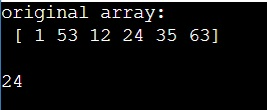# Find the kth maximum element in a NumPy array

Learn, how to find the kth maximum element in a NumPy array?
Submitted by Pranit Sharma, on March 22, 2023

## NumPy Array: Finding the kth maximum element

For example, if we are given an array [1,2,3,4,5] and we need the third maximum element in this array i.e., 3.

To find the kth maximum element in a NumPy array, we can just sort the numpy array and index the array with k-1 and the result will be the kth maximum element.

Let us understand with the help of an example,

## Python code to find the kth maximum element in a NumPy array

```# Import numpy
import numpy as np

# Creating a numpy array
arr = np.array([1,53,12,24,35,63])

# Display original array
print("original array:\n",arr,"\n")

# Defining k
k = 3

# Finding 3rd maximum element
res = arr[np.argsort(arr)][k-1]

# Display result
print(res)
```

### Output